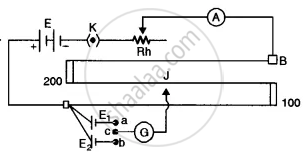# Draw a Labelled Circuit Diagram of a Potentiometer to Compare Emfs of Two Cells. Write the Working Formula (Derivation Not Required). - Physics (Theory)

Diagram

Draw a labelled circuit diagram of a potentiometer to compare emfs of two cells. Write the working formula (Derivation not required).

#### Solution

The required circuit diagram is given alongside.
Working formula "E"_1/"E"_2 = l_1/l_2Concept: Potentiometer
Is there an error in this question or solution?